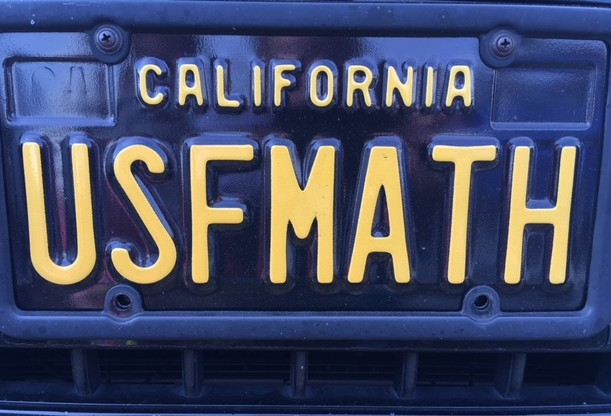Mathematics

Article

2012

Abstract

In this paper we consider the linear stability of a family of exact collapsing similarity solutions to the aggregation equation ρ t = ∇ · (ρ∇K * ρ) in Rd , d ⩾ 2, where K(r) = r γ/γ with γ > 2. It was previously observed [Y. Huang and A. L. Bertozzi, “Self-similar blowup solutions to an aggregation equation in Rn,” J. SIAM Appl. Math.70, 2582–2603 (Year: 2010)]10.1137/090774495 that radially symmetric solutions are attracted to a self-similar collapsing shell profile in infinite time for γ > 2. In this paper we compute the stability of the similarity solution and show that the collapsing shell solution is stable for 2 < γ < 4. For γ > 4, we show that the shell solution is always unstable and destabilizes into clusters that form a simplex which we observe to be the long time attractor. We then classify the stability of these simplex solutions and prove that two-dimensional (in-)stability implies n-dimensional (in-)stability.

Copyright 2012 American Institute of Physics. This article may be downloaded for personal use only. Any other use requires prior permission of the author and the American Institute of Physics.

The following article appeared in Sun, Hui, David Uminsky, and Andrea L. Bertozzi. 2012. "Stability and clustering of self-similar solutions of aggregation equations." Journal Of Mathematical Physics 53, no. 11. and may be found at http://dx.doi.org/10.1063/1.4745180.

DOI

10.1063/1.4745180

COinS